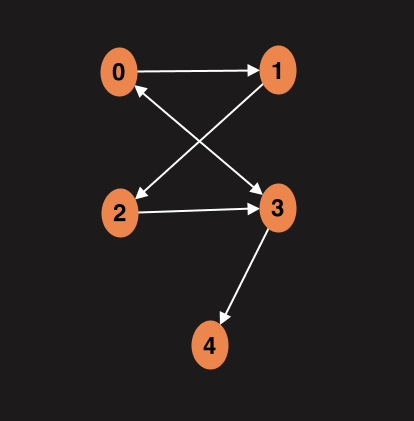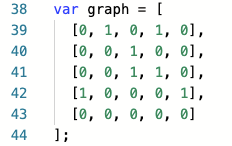1629045360

# JavaScript Algorithms and Data Structures: Graphs - Prim’s Algorithm

In computer science, Prim's algorithm is a greedy algorithm that finds a minimum spanning tree for a weighted undirected graph.

The algorithm operates by building this tree one vertex at a time, from an arbitrary starting vertex, at each step adding the cheapest possible connection from the tree to another vertex.Prim's algorithm starting at vertex A. In the third step, edges BD and AB both have weight 2, so BD is chosen arbitrarily. After that step, AB is no longer a candidate for addition to the tree because it links two nodes that are already in the tree.

## Minimum Spanning Tree

A minimum spanning tree (MST) or minimum weight spanning tree is a subset of the edges of a connected, edge-weighted (un)directed graph that connects all the vertices together, without any cycles and with the minimum possible total edge weight. That is, it is a spanning tree whose sum of edge weights is as small as possible. More generally, any edge-weighted undirected graph (not necessarily connected) has a minimum spanning forest, which is a union of the minimum spanning trees for its connected components.A planar graph and its minimum spanning tree. Each edge is labeled with its weight, which here is roughly proportional to its length.This figure shows there may be more than one minimum spanning tree in a graph. In the figure, the two trees below the graph are two possibilities of minimum spanning tree of the given graph.

## References

The Original Article can be found on https://github.com

#javascript #algorithms #datastructures #graphs

## Buddha Community1620466520

If you accumulate data on which you base your decision-making as an organization, you should probably think about your data architecture and possible best practices.

If you accumulate data on which you base your decision-making as an organization, you most probably need to think about your data architecture and consider possible best practices. Gaining a competitive edge, remaining customer-centric to the greatest extent possible, and streamlining processes to get on-the-button outcomes can all be traced back to an organization’s capacity to build a future-ready data architecture.

In what follows, we offer a short overview of the overarching capabilities of data architecture. These include user-centricity, elasticity, robustness, and the capacity to ensure the seamless flow of data at all times. Added to these are automation enablement, plus security and data governance considerations. These points from our checklist for what we perceive to be an anticipatory analytics ecosystem.

#big data #data science #big data analytics #data analysis #data architecture #data transformation #data platform #data strategy #cloud data platform #data acquisition1623479798

## Graph Data Structures and Algorithms in JavaScript for Beginners

Hello! Today I’ll be going over graphs . These data structures are the most widely used on the web, given the countless forms that a graph can organize values or even the data structures that can be made from them. In fact, the worldwide web itself can be represented as a graph. Let’s jump right into it!

#javascript #graph #algorithms #data-structures1596737580

## Graphs Data Structure: Breadth First Search

A week ago we learned about graph data structure. Today we will talk about how we can work with graphs. We will try to find distances between two nodes in a graph. This is one of the main uses of graphs and it’s called graph traversal. There are two main graph algorithms Breadth First Search (BFS) and Depth First Search (DFS) and today we will talk about BFS.

This is how our graph looks like:In our example we will work with an adjacency matrix. This is how matrix represents graph above:We will start with an input node, then visit all its neighbors which is one edge away. And then visit all their neighbors. Point is to determine how close the node is to the root node.

Function which we will write in a moment will return an object with key value pairs where key will represent node and value how far this node is from the root.

First we will loop over the adjacency matrix (2D array), create as many key value pairs as many nodes we have on the graph. Initially we will assign distance to the infinity which represents lack of connection between the nodes.

#graph #data-structures #breadth-first-search #javascript #algorithms #algorithms1597352100

## Graphs Data Structure: Depth First Search

In a previous blog post we talked about how to apply the Breadth First Search algorithm to the graph data structure. Today, let’s figure out how Depth First Search (DFS) works.

DFS is one of the fundamental algorithms used to search nodes and edges in a graph. It’s a form of a traversal algorithm.

Just a reminder, this is how our graph looks:Based on the name we can assume that BFS focuses on the depth of the graph. The search starts at some root node and it keeps searching as far as possible each branch before backtracking.

Our task is to write an algorithm that explores routes as deep as possible before going back and exploring other routes. Let’s see how we can do it.

#data-structures #graph #javascript #algorithms #algorithms1629045360

## JavaScript Algorithms and Data Structures: Graphs - Prim’s Algorithm

In computer science, Prim's algorithm is a greedy algorithm that finds a minimum spanning tree for a weighted undirected graph.

The algorithm operates by building this tree one vertex at a time, from an arbitrary starting vertex, at each step adding the cheapest possible connection from the tree to another vertex.Prim's algorithm starting at vertex A. In the third step, edges BD and AB both have weight 2, so BD is chosen arbitrarily. After that step, AB is no longer a candidate for addition to the tree because it links two nodes that are already in the tree.

## Minimum Spanning Tree

A minimum spanning tree (MST) or minimum weight spanning tree is a subset of the edges of a connected, edge-weighted (un)directed graph that connects all the vertices together, without any cycles and with the minimum possible total edge weight. That is, it is a spanning tree whose sum of edge weights is as small as possible. More generally, any edge-weighted undirected graph (not necessarily connected) has a minimum spanning forest, which is a union of the minimum spanning trees for its connected components.A planar graph and its minimum spanning tree. Each edge is labeled with its weight, which here is roughly proportional to its length.This figure shows there may be more than one minimum spanning tree in a graph. In the figure, the two trees below the graph are two possibilities of minimum spanning tree of the given graph.

## References

The Original Article can be found on https://github.com

#javascript #algorithms #datastructures #graphs